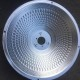Characteristics of the use of steel materials

(1) Melting point

°C
The melting temperature when a metal material changes from a solid state to a liquid state

(2) Specific heat capacity
c
J/(kg·K)
A certain substance per unit mass absorbs heat when the temperature is 1°C higher or releases heat when the temperature drops by 1°C

(3) Thermal conductivity
λ
W/(m·k)
In unit time, when the temperature drops by 1℃ per unit length along the direction of heat flow, the heat per unit area is allowed to conduct

(4) Linear expansion coefficient
αL
10‐⒍/K
The ratio of the increased length to the original length for every 1℃ increase in metal temperature

3.
Electricity
sex
can
(1) Resistivity
ρ
Ω˙m
It is a parameter indicating the electrical conductivity of an object. It is equal to the resistance between the ends of a wire with a length of 1 m and a cross-sectional area of ​​1 mm2. It can also be expressed by the resistance between two parallel end faces of a unit cube

(2) Temperature coefficient of resistance
αρ
1/℃
The ratio of the change of material resistivity to the original resistivity for every 1℃ temperature rise and fall is called the temperature coefficient of resistance

(3) Conductivity
k
S/m or %IACS
The reciprocal of electrical resistance is called conductivity. In terms of value, it is equal to the current flowing through a unit area when the conductor maintains a unit elevator degree.

4.
magnetic
sex
can
(1) Magnetic permeability
μ
H/m
It is a performance index to measure the difficulty of magnetization of a magnetic material. It is the ratio of the magnetic induction reaction intensity (B) and the magnetic field intensity (H) in the magnetic material. Magnetic materials are usually divided into two categories: soft magnetic materials (μ) with very high value, up to tens of thousands) and hard magnetic materials (μ value is on the right of 1)

(2) Magnetic induction intensity
B
T
The magnetization process in the magnetic medium can be regarded as adding a new magnetic field determined by the magnetization (J) to the original magnetic field intensity (H), the number is equal to 4πJ, so the magnetic field in the magnetic medium B= H+4πJ, called the magnetic induction intensity

(3) Magnetic field hardness
H
A/m
Electric current flows through the conductor, and a magnetic field is generated around it. The force of the magnetic field on the original magnetic moment or current is a sign of the magnetic field strength

(4) Coercivity
He
A/m
After the sample is magnetized to saturation, due to the hysteresis phenomenon, the bath reduces the magnetic induction intensity to 0, and a certain negative magnetic field He and Hc must be applied, which is called the coercive force.

(5) Iron loss
р
W/kg
The energy consumed by ferromagnetic materials due to hysteresis and eddy current effects under dynamic magnetization conditions

When a metal sample is stretched, the ratio of the maximum load to the original cross-sectional area of ​​the sample before it breaks is called the tensile strength бb=Pb/Fo
Where Pb—the maximum load before the sample is broken (N)
Fo—The original cross-sectional area of ​​the sample (mm2)

(2) Flexural strength
бbb
MPa

When the sample is broken under the action of the concentrated load located in the middle of the two supports, the maximum pressure that the fractured section will bear against the round sample: бbb=8PL/πd3
For rectangular specimens: бbb=3PL /2bh2
In the formula, P—-maximum concentrated load on the sample (N)
L—-Span between two supporting points (mm)
d—Outer diameter of round sample section (mm)
b-Width of rectangular cross-section sample (mm)
h—The height of the rectangular section specimen (mm)

(3) Compressive strength
бbc
MPa

The maximum positive pressure that a material can withstand without breaking or cracking under pressure is called compressive strength
бbc=Pbc/F o
Where Pbc—the maximum concentrated load on the sample (N)
F o—Sectional area of ​​sample analysis (mm2)

(4) Shear strength
τστ
MPa

Before the sample is cut, the average shear stress of the shear section under the maximum load
Double shear: στ=P/2Fo; single shear: στ=P/Fo
Where P—maximum load during shearing (N)
F o—the original cross-sectional area of ​​the sheared part (mm2)

(5) Torsional strength
τ
MPa

Refers to the external force is the strength limit of the torsion force
τb≈3Mb/4Wp (applicable to steel)
τb≈Mb/Wp (applicable to cast iron)
Where Mb—torque moment (N•)
Wp—The polar section coefficient of the sample section during torsion (mm2)

(6) Yield point
σs
MPa

During the pulling process of the metal sample, the load no longer increases, and the phenomenon that the sample continues to deform is called “yield”. The stress at which the yield phenomenon occurs, called the yield point or yield limit
σs=Ps/Fo
Fo—The original cross-sectional area of ​​the sample (mm2)

(7) Yield strength
σ0.2
MPa

For some metal materials whose yield phenomenon is not obvious, it is difficult to determine the yield point. The stress that produces 0.2% permanent deformation is often defined as the yield point, which is called the yield strength or the conditional yield limit.
σ0.2P0.2/Fo
In the formula P0.2—the load when the sample has a permanent deformation of 0.2% (N)
Fo—The original cross-sectional area of ​​the sample (mm2)

(8) Durable strength
σb/time (h)<